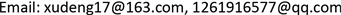﻿ 几类n2阶BCCB哈达码矩阵的构造 On the Construction of Several Types of BCCB Complex Hadamard Matrices of Order n2

Pure Mathematics
Vol. 08  No. 06 ( 2018 ), Article ID: 27594 , 6 pages
10.12677/PM.2018.86088

On the Construction of Several Types of BCCB Complex Hadamard Matrices of Order n2

Dengming Xu1, Qianqian Yan2

1Sino-European Institute of Aviation Engineering, Civil Aviation University of China, Tianjin

2College of Science, Civil Aviation University of China, TianjinReceived: Oct. 24th, 2018; accepted: Nov. 5th, 2018; published: Nov. 16th, 2018ABSTRACT

In this note, we study how to construct BCCB complex Hadamard matrices. We first give a necessary and sufficient condition for a BCCB complex matrix of order n2 to be Hadamard, and then use the condition to construct various types of BCCB complex Hadamard matrices. As an example, three new types of BCCB complex Hadamard matrices of order 16 are provided.

Keywords:Circulant Matrices, Circulant Matrices with Circulant Blocks, Hadamard Matrices

1中国民航大学中欧航空工程师学院，天津

2中国民航大学理学院，天津1. 引言

2. 预备知识

$n\ge 2$ 是自然数。

1) 对角阵 $D=diag\left({a}_{0},{a}_{1},\cdots ,{a}_{n-1}\right)$ 定义如下：

$\forall 1\le i,j\le n,\text{\hspace{0.17em}}\text{\hspace{0.17em}}{D}_{jk}={\delta }_{jk}{a}_{k-1}$ .

2) 循环阵 $C=Circ\left({a}_{0},{a}_{1},\cdots ,{a}_{n-1}\right)$ 定义如下：

$\forall 1\le j,k\le n,\text{\hspace{0.17em}}\text{\hspace{0.17em}}{C}_{j,k}={a}_{\left(n-1\right)j\oplus k}$

$C=\left(\begin{array}{ccccc}{a}_{0}& {a}_{1}& {a}_{2}& \cdots & {a}_{n-1}\\ {a}_{n-1}& {a}_{0}& {a}_{1}& \cdots & {a}_{n-2}\\ {a}_{n-2}& {a}_{n-1}& {a}_{0}& \cdots & {a}_{n-3}\\ \cdots & \cdots & \cdots & \ddots & \cdots \\ {a}_{1}& {a}_{2}& {a}_{3}& \cdots & {a}_{0}\end{array}\right)$

3) n2阶方阵C被称为由循环块组成的循环矩阵(BCCB矩阵)是指它具有下面形式：

$C=Circ\left({C}_{0},{C}_{1},\cdots ,{C}_{n-1}\right)$

4) 一个n阶方阵H被称为复哈达码矩阵是指：方阵中每个元素的模都是1，并且 ${H}^{*}H={I}_{n}$ ，其中， ${I}_{n}$ 是n阶单位阵，*是厄米特转置。

${\omega }_{n}={\text{e}}^{\frac{2\pi i}{n}}$ ，傅立叶矩阵 ${F}_{n}$ 定义如下：

$\forall 1\le i,j\le n,\text{\hspace{0.17em}}\text{\hspace{0.17em}}{\left({F}_{n}\right)}_{jk}={\omega }^{\left(j-1\right)\left(i-1\right)}$

${D}_{n}=diag\left(1,{\omega }_{n},{\omega }_{n}^{2},\cdots ,{\omega }_{n}^{n-1}\right),\text{\hspace{0.17em}}\text{\hspace{0.17em}}{J}_{n}=Circ\left(0,1,0,\cdots ,0\right)$

1) ${U}_{n}^{-1}{J}_{n}{U}_{n}={D}_{n}$

2) 若 $C=Circ\left({a}_{0},{a}_{1},\cdots ,{a}_{n-1}\right)$${P}_{v}={a}_{0}+{a}_{1}X+\cdots +{a}_{n-1}{X}^{n-1}$ ，则

${U}_{n}^{-1}C{U}_{n}=diag\left({P}_{v}\left(1\right),{P}_{v}\left({\omega }_{n}\right),\cdots ,{P}_{v}\left({\omega }_{n}^{n-1}\right)\right)$

3. 矩阵的构造及例子

$C={I}_{n}\otimes {C}_{0}+{J}_{n}\otimes {C}_{1}+\cdots +{J}_{n}^{n-1}\otimes {C}_{n-1}$ .

${U}^{-1}CU={I}_{n}\otimes {U}_{n}^{-1}{C}_{0}{U}_{n}+{D}_{n}\otimes {U}_{n}^{-1}{C}_{1}{U}_{n}+\cdots +{D}_{n}^{n-1}\otimes {U}_{n}^{-1}{C}_{n-1}{U}_{n}$ .

${V}_{k}={U}_{n}^{-1}{C}_{k}{U}_{n}=diag\left({P}_{k}\left(1\right),{P}_{k}\left({w}_{n}\right),{P}_{k}\left({w}_{n}^{2}\right),\cdots ,{P}_{k}\left({w}_{n}^{n-1}\right)\right)$ ,

${W}_{k}={V}_{0}+{\omega }_{n}^{k}{V}_{1}+{\omega }_{n}^{2k}{V}_{2}+\cdots +{\omega }_{n}^{k\left(n-1\right)}{V}_{n-1}$ .

${U}^{-1}CU=diag\left({W}_{0},{W}_{1},\cdots ,{W}_{n-1}\right)$ 。易知对任意 $0\le k\le n-1,1\le j\le n$

${\left({W}_{k}\right)}_{j,j}=\left(1,{\omega }_{n}^{k},{\omega }_{n}^{2k},\cdots ,{\omega }_{n}^{k\left(n-1\right)}\right)A\left(1,{\omega }_{n}^{j-1},{\omega }_{n}^{2\left(j-1\right)},\cdots ,{\omega }_{n}^{\left(j-1\right)\left(n-1\right)}\right)$

$\forall 0\le k\le n-1,\text{\hspace{0.17em}}\forall 1\le j\le n,\text{\hspace{0.17em}}\text{\hspace{0.17em}}|{\left({W}_{k}\right)}_{j,j}|=n$ .

${F}_{3}^{*}D=\left(\begin{array}{ccc}a& b& c\\ a& b\omega & c{\omega }^{2}\\ a& b{\omega }^{2}& c\omega \end{array}\right)$

1) 若V是对角矩阵，则 $\text{BCCB}\left(V{F}_{n}\right)$$\text{BCCB}\left({F}_{n}V\right)$ 是哈达码矩阵。

2) 若 ${U}_{n}V{U}_{n}^{*}$ 中元素模全为1，则 $\text{BCCB}\left(V\right)$ 是哈达码矩阵。

3) 若 ${F}_{n}V=V{F}_{n}$ ，则 $\text{BCCB}\left(V\right)$ 是哈达码矩阵。

1) 因为 ${U}_{n}$ 是酉矩阵，故  。因此 ${U}_{n}V{F}_{n}{U}_{n}$ 中元素模全为1。由定理3.1知 $\text{BCCB}\left(V{F}_{n}\right)$ 是哈达码矩阵。同理可证 $\text{BCCB}\left({F}_{n}V\right)$ 也是哈达码矩阵。

2) 首先， ${U}_{n}V{U}_{n}={U}_{n}V{U}_{n}^{*}{U}_{n}^{2}={U}_{n}V{U}_{n}^{*}P$ 。因为 ${U}_{n}V{U}_{n}^{*}$ 中元素模全为1，故 ${U}_{n}V{U}_{n}$ 中元素模全为1。由定理3.1知 $\text{BCCB}\left(V\right)$ 是哈达码矩阵。

3) 由 ${F}_{n}V=V{F}_{n}$${U}_{n}V=V{U}_{n}$ ，于是有 ${U}_{n}V{U}_{n}=V{U}_{n}^{2}=VP$ ，故 ${U}_{n}V{U}_{n}$ 中元素模全为1。由定理3.1知 $\text{BCCB}\left(V\right)$ 是哈达码矩阵。□

${R}_{4}=\frac{1}{2}\left(\begin{array}{cccc}1& \sqrt{2}& 1& 0\\ 1& 0& -1& \sqrt{2}\\ -1& \sqrt{2}& -1& 0\\ 1& 0& -1& -\sqrt{2}\end{array}\right)$

$A={R}_{4}{D}_{4}{R}_{4}^{*}=\frac{1}{4}\left(\begin{array}{cccc}2b+a+c& a-c& 2b-\left(a+c\right)& a-c\\ a-c& a+c+2d& c-a& a+c-2d\\ 2b-\left(a+c\right)& c-a& 2b+a+c& c-a\\ a-c& a+c-2d& c-a& a+c+2d\end{array}\right)$

$\left\{\begin{array}{l}|a+c±2b|=4\\ |a+c±2d|=4\\ |a-c|=4\end{array}$ (1)

$A=\left(\begin{array}{cccc}{\text{e}}^{i{\theta }_{1}}& {\text{e}}^{i{\theta }_{2}}& {\text{e}}^{i{\theta }_{2}}& {\text{e}}^{i{\theta }_{1}}\\ {\text{e}}^{i{\theta }_{1}}& {\text{e}}^{i{\theta }_{3}}& -{\text{e}}^{i{\theta }_{1}}& -{\text{e}}^{i{\theta }_{3}}\\ {\text{e}}^{i{\theta }_{2}}& -{\text{e}}^{i{\theta }_{1}}& {\text{e}}^{i{\theta }_{2}}& -{\text{e}}^{i{\theta }_{1}}\\ {\text{e}}^{i{\theta }_{1}}& -{\text{e}}^{i{\theta }_{3}}& -{\text{e}}^{i{\theta }_{1}}& {\text{e}}^{i{\theta }_{3}}\end{array}\right)$

$\left\{\begin{array}{l}|a+c+2b|=4\\ |a+c-2b|=4\\ |a-c|=4\end{array}$ (2)

i) 假设 $b=d$ 。由方程组(2)知，存在 $\left({\theta }_{1},{\theta }_{2},{\theta }_{3}\right)\in {ℝ}^{3}$ 使得

$\left\{\begin{array}{l}a+c+2b=4{\text{e}}^{i{\theta }_{1}}\\ a+c-2b=4{\text{e}}^{i{\theta }_{2}}\\ a-c=4{\text{e}}^{i{\theta }_{3}}\end{array}$

$A=\left(\begin{array}{cccc}{\text{e}}^{i{\theta }_{1}}& {\text{e}}^{i{\theta }_{2}}& {\text{e}}^{i{\theta }_{3}}& {\text{e}}^{i{\theta }_{2}}\\ {\text{e}}^{i{\theta }_{2}}& {\text{e}}^{i{\theta }_{1}}& -{\text{e}}^{i{\theta }_{2}}& -{\text{e}}^{i{\theta }_{3}}\\ {\text{e}}^{i{\theta }_{3}}& -{\text{e}}^{i{\theta }_{2}}& {\text{e}}^{i{\theta }_{1}}& -{\text{e}}^{i{\theta }_{2}}\\ {\text{e}}^{i{\theta }_{2}}& -{\text{e}}^{i{\theta }_{3}}& -{\text{e}}^{i{\theta }_{2}}& {\text{e}}^{i{\theta }_{1}}\end{array}\right)$

ii) 假设 $b=-d$ 。同理，对任意 $\left({\theta }_{1},{\theta }_{2},{\theta }_{3}\right)\in {ℝ}^{3}$ ，若取

$A=\left(\begin{array}{cccc}{\text{e}}^{i{\theta }_{1}}& {\text{e}}^{i{\theta }_{2}}& {\text{e}}^{i{\theta }_{3}}& {\text{e}}^{i{\theta }_{2}}\\ {\text{e}}^{i{\theta }_{2}}& -{\text{e}}^{i{\theta }_{3}}& -{\text{e}}^{i{\theta }_{2}}& {\text{e}}^{i{\theta }_{1}}\\ {\text{e}}^{i{\theta }_{3}}& -{\text{e}}^{i{\theta }_{2}}& {\text{e}}^{i{\theta }_{1}}& -{\text{e}}^{i{\theta }_{2}}\\ {\text{e}}^{i{\theta }_{2}}& {\text{e}}^{i{\theta }_{1}}& -{\text{e}}^{i{\theta }_{2}}& -{\text{e}}^{i{\theta }_{3}}\end{array}\right)$

On the Construction of Several Types of BCCB Complex Hadamard Matrices of Order n2[J]. 理论数学, 2018, 08(06): 656-661. https://doi.org/10.12677/PM.2018.86088

1. 1. Caidman, L., Aharonov, Y. and Albert, D.Z. (1987) How to Ascertain the Values of σx, σy and σz of a Spin-1/2 Particle. Physical Review Letters, 58, 1385-1387.
https://doi.org/10.1103/PhysRevLett.58.1385

2. 2. Englert, B.G. and Aharonov, Y. (2001) The Mean King’s Problem: Prime Degrees of Freedom. Physics Letters A, 284, 1-5.
https://doi.org/10.1016/S0375-9601(01)00271-7

3. 3. Bandyopadhyay, S., Boykin, P.O., Roychowdhury, V. and Vatan, F. (2002) A New Proof for the Existence of Mutually Unbiased Bases. Algorithmica, 34, 512-528.
https://doi.org/10.1007/s00453-002-0980-7

4. 4. Brierley, S. (2009) Mutually Unbiased Bases in Low Dimensions. PhD Thesis, University of York, York.

5. 5. Wootters, W.K. and Fields, B.D. (1989) Optimal State-Determination by Mutually Unbiased Meas-urements. Annals of Physics, 191, 363-381.
https://doi.org/10.1016/0003-4916(89)90322-9

6. 6. Liu, J.Y., Yang, M.H. and Feng, K.Q. (2017) Mutually Unbiased Maximally Entangled Bases in . Quantum Information Processing, 16, 159.
https://doi.org/10.1007/s11128-017-1608-9

7. 7. Tao, Y.H., Nan, H, Zhang, J. and Fei, S.M. (2015) Mutually Unbiased Maxi-mally Entangled Bases in . Quantum Information Processing, 14, 2291-2300.
https://doi.org/10.1007/s11128-015-0980-6

8. 8. Xu, D.M. (2017) Construction of Mutually Unbiased Maximally Entangled Bases through Permutations of Hadamard Matrices. Quantum Information Processing, 16, 65.
https://doi.org/10.1007/s11128-017-1534-x

9. 9. Tadej, W. and Zyczkowski, K. (2006) A Concise Guide to Complex Hadamard Matrices. Open Systems and Information Dynamics, 13, 133-177.
https://doi.org/10.1007/s11080-006-8220-2

10. 10. Combescure, M. (2009) Block-Circulant Matrices with Circulant Blocks, Weil Sums, and Mutually Un-Biased Bases. II. The Prime Power Case. Journal of Mathematical Physics, 50, Article ID: 032104.
https://doi.org/10.1063/1.3078420

11. 11. Karlsson, B.R. (2016) BCCB Complex Hadamard Matrices of Order 9, and MUBs. Linear Algebra and Its Applications, 501, 309-324.
https://doi.org/10.1016/j.laa.2016.04.012

12. 12. Davis, P.J. (1979) Circulant Matrices. John Wiley and Sons, New York.